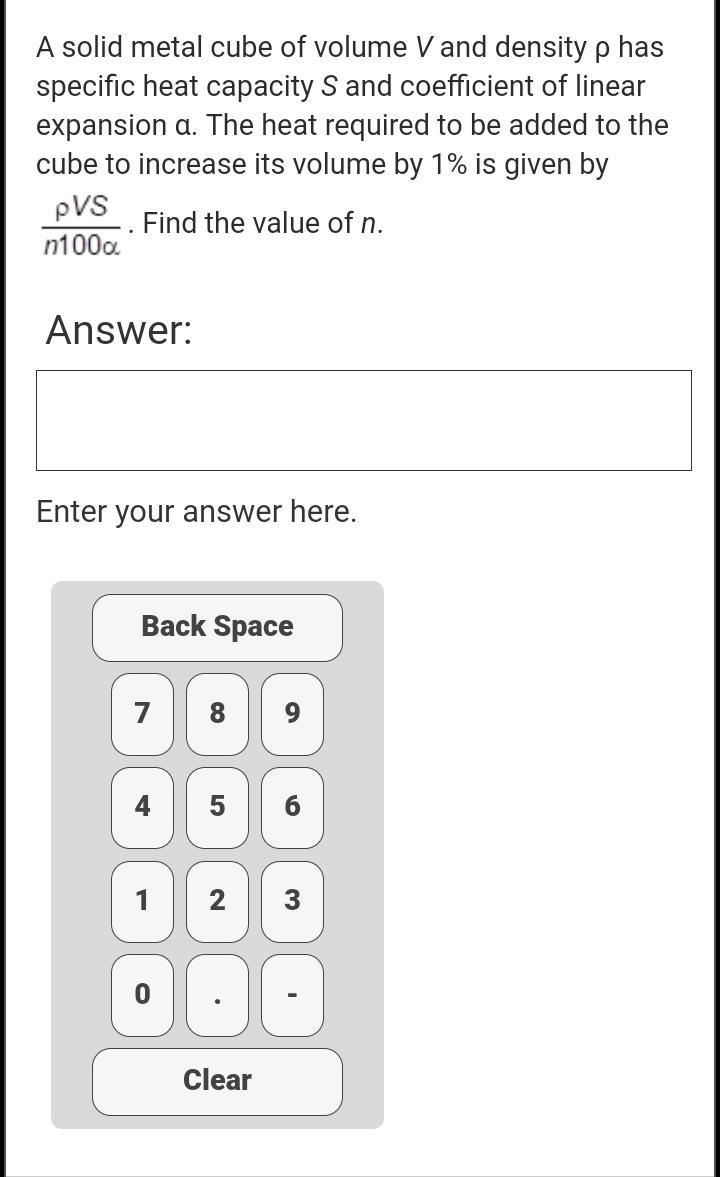Question:

# A solid metal cube of volume V and density p has specific heat capacity S and coefficient of linear expansion a. The heat requirA solid metal cube of volume V and density p has specific heat capacity S and coefficient of linear expansion a. The heat required to be added to the cube to increase its volume by 1% is given by PVS Find the value of n. n100a . Answer: Enter your answer here. Back Space 7 8 9 5 6 1 N 3 . Clear